# Originally given in December 1991

This exam consists of 2 parts. Part I contains 10 short ``grab-bag'' questions, each worth 4 points. Part II contains 5 longer problems, each worth 12 points. The exam was closed-book, but allowed a crib sheet. Unlike this year, a non-graphics calculator was allowed.

Evaluate the following derivatives, integrals, and limits, and simplify the following expressions involving exponentials and logs.

For problems A-D, you do NOT have to reduce your answers to the simplest possible forms. Any correct answers will do.

However, for problems E-J, I do want answers in the simplest possible form. For example, if the answer is zero, then writing ``ln(1)'' (which DOES equal zero, of course) will only get you partial credit. Also, you should show your reasoning. Just pulling numbers from a calculator will get you NO credit.

Some of the limits may not exist. If they don't exist, write ``DNE'' or ``does not exist'' in the box.

A. Find f'(x), where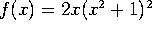.

B. Evaluate: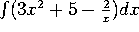.

C. Find f'(x), where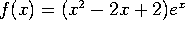D. Find f'(x), where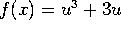and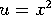.

E. Evaluate (and simplify):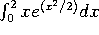F. Evaluate (and simplify):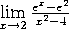G. Evaluate (and simplify):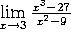H. Simplify: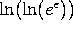I. Simplify: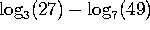.

J. Find dy/dx at the point (2,3), where y is given by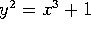. (Your answer should be a NUMBER, not something like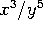.)

This part consists of 5 longer problems, each worth 12 points.

Problem 1. A driver goes out on a 4-hour car trip. v(t), his velocity at time t, is given by the formula: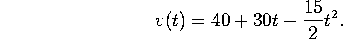Here t is measured in hours, and v is measured in miles/hour.

Note that parts (a) and (b) below can be done independently of each other. Part (a) involves differentiation, while part (b) involves integration.

a) At what time is the driver driving the fastest? How fast is he going at that time?

b) How far does the driver travel in 4 hours?

Problem 2. Consider the function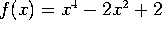.

a) Find all the local maxima of f(x), all the local minima, and all the points of inflection.

b) For what values of x is f increasing?

c) For what values of x is the graph of f concave up?

Problem 3. C-14, an isotope of carbon, is radioactive. Suppose the radiation from a sample of C-14 decreases at a rate of 0.014% per year, compounded continuously (this figure is pretty accurate).

a) What is the half-life of C-14 (in other words, how long do you have to wait for half the radioactivity to be gone)?

b) If 1 pound of C-14 were deposited 2000 years ago, how much of it would be left today?

c) If a sample of ancient wood contains only one tenth of the C-14 that it used to, how old is it?

4. a) Use differentials to estimate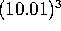.

b) The radius of a ball is increasing at a rate of 1 ft/sec. At what rate is the volume increasing when the radius reaches 10 feet? (Hint: the volume of a sphere of radius r is given by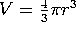).

5. Consider the function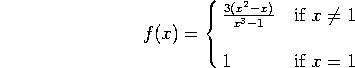a) Does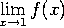exist? Why or why not? If it exists, what does it equal?

b) Is f(x) continuous at x=1? Why or why not?